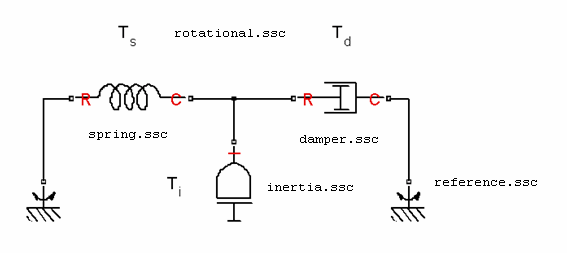## Declare a Spring Component

The following diagram shows a network representation of a mass-spring-damper system, consisting of four components (mass, spring, damper, and reference) in a mechanical rotational domain.The domain is declared in a file named `rotational.ssc` (see Declare a Mechanical Rotational Domain). The following file, named `spring.ssc`, declares a component called spring. The component contains:

• Two rotational nodes, `r` and `c` (for rod and case, respectively)

• Parameter `k`, with a default value of `10 N*m/rad`, specifying the spring rate

• Through and Across variables, torque `t` and angular velocity `w`, later to be related to the Through and Across variables of the rotational domain

• Internal variable `theta`, with a default value of `0 rad`, specifying relative angle, that is, deformation of the spring

```component spring nodes r = foundation.mechanical.rotational.rotational; c = foundation.mechanical.rotational.rotational; end parameters k = { 10, 'N*m/rad' }; % spring rate end variables theta = { 0, 'rad' }; % introduce new variable for spring deformation t = { 0, 'N*m' }; % torque through w = { 0, 'rad/s' }; % velocity across end % branches here % equations here end ```

Note

This example shows only the declaration section of the spring component. For a complete file listing of a spring component, see Mechanical Component — Spring.